MORE IN Circuits and Transmission Lines
MU Electronics and Telecom Engineering (Semester 3)
Circuits and Transmission Lines
December 2015
Total marks: --
Total time: --
INSTRUCTIONS
(1) Assume appropriate data and state your reasons
(2) Marks are given to the right of every question
(3) Draw neat diagrams wherever necessary

1 (a) Determine the ABCD parameter of the network shown in fig. No. 1(a)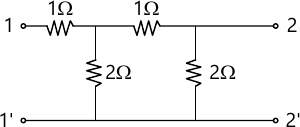5 M
1 (b) Test whether P(s)=S5+12S4+45S3+60S2+44S+48 is Hurwitz polynomial.
5 M
1 (c) The combined inductance of two coils connected in series is 0.6 H or 0.1 H depending on relative directions of currents in the two coils. If one of the coil has a self inductance of 0.2 H. Find (a) Mutual inductance (b) Coefficient of coupling.
5 M
1 (d) Find Foster I and II Cauer I and II Circuits for the driving points admittance $$y(s) = \dfrac {s^2+1}{s}$$
5 M

2 (a) Find the current in the 10Ω resistor using Thevenin's theorem for the network shown in fig 2(a).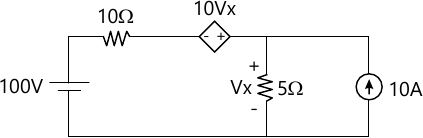10 M
2 (b) Find the value of Vx in the network shown in fig 2(b) using nodal analysis.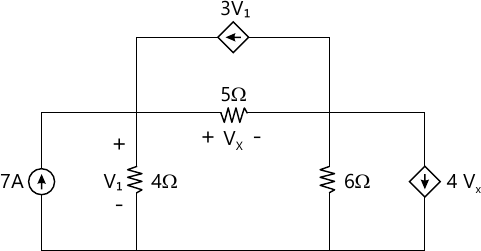5 M
2 (c) Check if the following polynomials are Hurwitz polynomials
i) S5 + S3 + S
ii) S4 + S3 + 2S2 + 3S +2.
5 M

3 (a) Synthesize the driving point function $$F(s) = \dfrac {(S^2 +1)(S^2+3)}{S(S^2+2)}$$ when F(s) is a driving point (i) Impedance (ii) Admittance
Test if the circuit obtained are canonic:
10 M
3 (b) State and prove initial value theorem.
5 M
3 (c) The parameters of a transmission line are R=6Ω/km, L=2.2 mH/km G=0.25 ×10-6 ℧/km, C=0.005 × 10-6 F/km. Determine the characteristics impedance and propagation constant at a frequency of 1 GHz.
5 M

4 (a) Determine z and y parameters of the network shown in fig 4(a).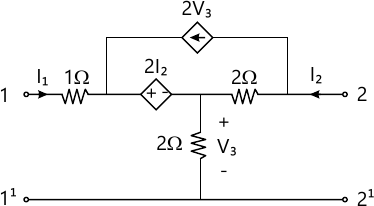10 M
4 (b) Determine the voltage transfer function $$\dfrac {v_2}{v_1}$$ for the network shown in fig 4(b).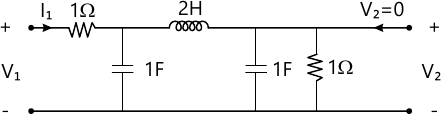5 M
4 (c) Test whether $$F(s)= \dfrac {2S^3 + 2S^2 + 3S+2}{S^2+1}$$ is a positive Real Function.
5 M

5 (a)

The network shown in fig 5(a), a steady state is reached with the switch open. At t=0 the switch is closed. Determine VC(0-), i1(0+), i2(0+), $$\dfrac {di_1}{dt}(0^+) \text{ and }\dfrac {di_2}{dt}(0^+)$$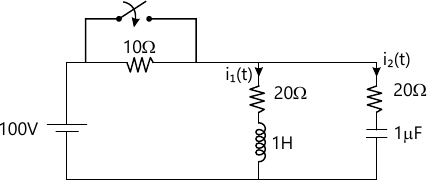10 M
5 (b) Find the voltage across the 5Ω resistor in network shown in fig, 5(b).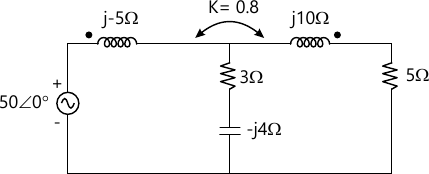5 M
5 (c) Find the function v(t) using the pole-zero of the following function. $$V(s) = \dfrac {(S+2)(S+6)}{(S+1)(S+5)}$$.
5 M

6 (a) A unit impulse applied to two terminal black box procedure a voltage VO(t)=2e-t-e-3t. Determine the terminal voltage when a current pulse of 1A heigh and a duration of 2 seconds in applied at the terminal.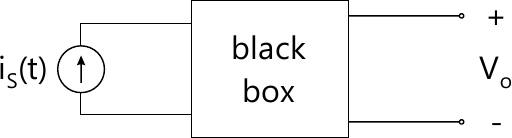10 M
6 (b) Determine the driving point impedance of the network shown in fig. 6(b)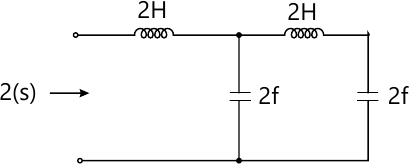5 M
6 (c) Draw the following normalized quantities on the smith chart:
(2 + j2)Ω
ii) (4-j2)Ω
iii) (1,0)Ω
iv) (j1, 0)Ω
5 M

More question papers from Circuits and Transmission Lines